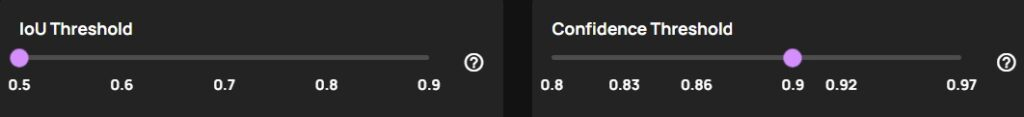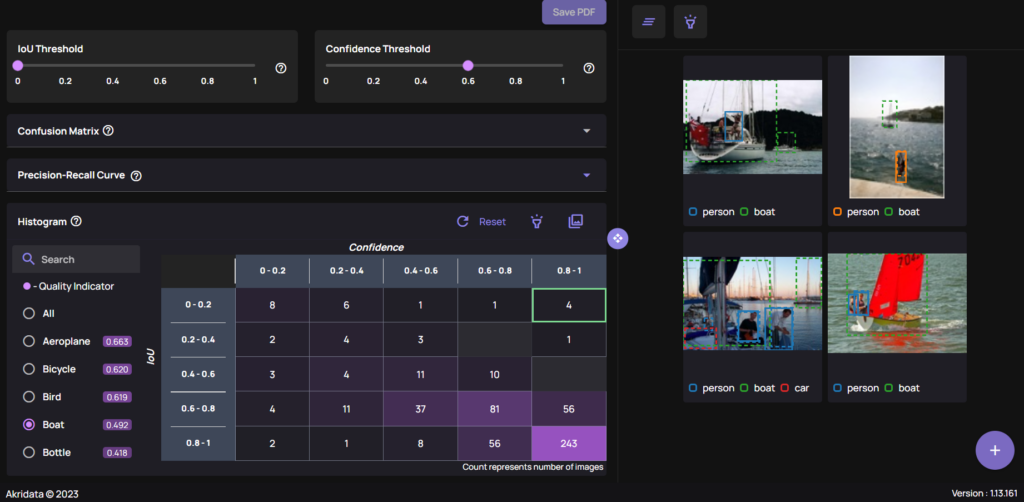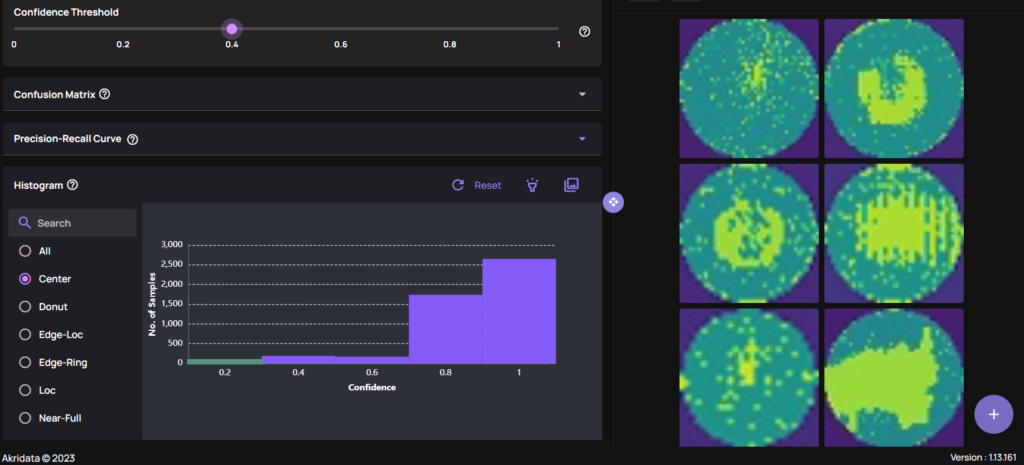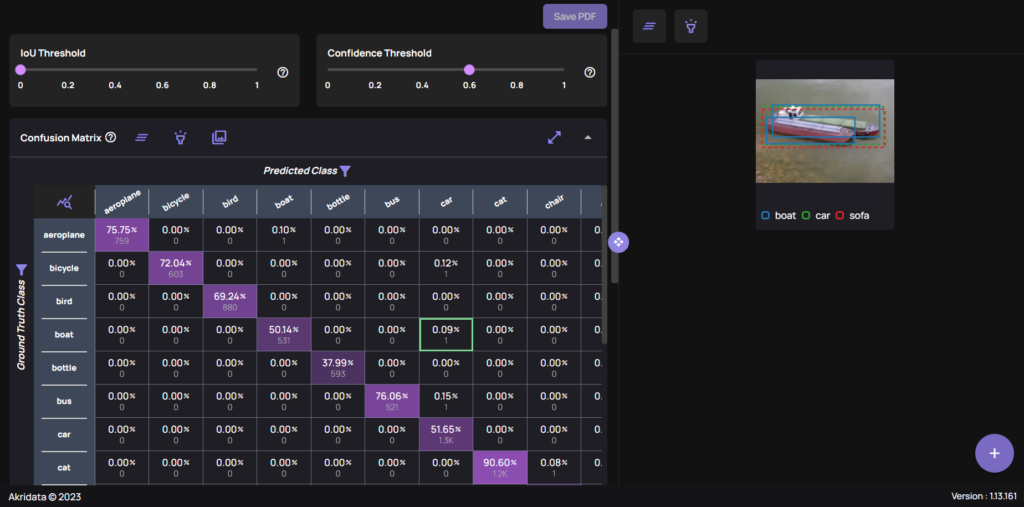Akridata

# Modern Model Accuracy Analysis## Introduction

Improving a model’s accuracy is a tricky task, with an open question — where do you focus your resources?
The data-centric AI approach focuses on the data — improve the data quality where needed, focus the training cycles where the model suffers and you should be on the right path. But how do you identify these pain points?

Confusion matrices, P-R curves, accuracy histograms and other metrics are standard practices in determining a model’s accuracy. Each one provides a slice through the model and the data, emphasizing potential key points to focus on.

Can we go one step forward? YES!

## Modern accuracy analysis

Akridata’s Data Explorer offers an interactive platform, where the accuracy metrics are connected directly to the data, saving valuable time in analyzing model’s accuracy, understanding what caused inaccuracies and allowing DS teams to target the next training cycle exactly where the model misfires.

## Classification, Object Detection, Segmentation

Data Explorer is an AI platform that saves hours on visual data curation and lowers overall development costs, by giving the researchers and data scientists a simple way to manage their data, reducing annotation spend and eliminating wasted training cycles.

For each branch of computer vision — classification, object detection and segmentation, Data Explorer provides accuracy metrics, that are interactively controlled by relevant parameters and offer an immediate insight into the data behind them.

IOU & Confidence Threshold

• IOU Threshold
When comparing two bounding boxes, typically these are the labeled and the predicted, we compare their intersection area with their combined area, or, Intersection divided by the Union (IOU). This value measures how similar the bounding boxes are, and can be used for comparing segmentation masks too. A threshold can be set, to ignore all predicted bounding boxes whose IOU value is too low — this will be the IOU Threshold.
• Confidence Threshold
The Confidence Score is a value that represents the model’s confidence in its prediction. If we set a threshold on this parameter, we can remove all the predictions a model isn’t certain about — this is the Confidence Threshold.

The platform allows us to control the IOU Threshold and the Confidence Threshold at different granularities. By default we get a uniform distribution across the [0, 1] range for either threshold, as seen below:###### Uniform IOU and Confidence Threshold values.

However, if a specific range is more suitable, that can be set, as seen below:###### IOU and Confidence Threshold custom range & values.

Both thresholds are relevant for Object Detection and Segmentation problems, while for the Classification case only the Confidence Threshold plays a role.

Precision-Recall Curve

A Precision-Recall Curve plots the trade-off between precision and recall at different thresholds, and is a useful measure when prioritizing the number of false positives or capturing as many true positives as possible.

A PR-Curve for each object is provided, where we see how its shape is affected by choosing a different threshold, allowing the DS team to balance between Precision and Recall values. A PR-Curve for “Bird” is displayed below:###### A PR-Curve for “Bird”, based on selected threshold.

A PR-Curve is provided for all problem types — Classification, Object Detection and Segmentation.

Confidence — IOU Histogram

A different way of slicing a model’s accuracy, is to look at the Confidence-IOU histogram. For each object, we can see the number of elements in a specific cell, as in the example below:###### Confidence-IOU Histogram. Each cell is linked to the images.

Examples in the top left corner may flush-out a problem — a model’s output with high confidence, but a low IOU might indicate a serious accuracy concern, or an error on the labeling part. Below is an example that demonstrates this — top right cell of the histogram contains four instances of model predictions with a high Confidence score (> 0.8), while a very low IOU with labeled data (< 0.2).

When the images related to the cell are viewed, each shows examples of missing labels, as seen on the right of the image.###### (Left) Top right of Confidence-IOU histogram contains examples of model predictions with high Confidence but low IOU. (Right) The corresponding images to the cell all contain missing labels.

A Confidence-IOU histogram is provided for Object Detection and Segmentation problems, while in the Classification case, a bar plot of Confidence vs Number of Samples is shown. As seen below, the bar plot is shown per class, and similar to the histogram above, each bin provides direct access to the images:###### Classification problem: Bar plot of Confidence vs Number of Samples. Each bin is linked to the images.

Confusion Matrix

A confusion matrix visualizes a model’s accuracy, counting the number of correct and incorrect predictions, given certain IOU & Confidence thresholds.

As with the histogram above, each cell in the confusion matrix can be clicked to view the instances it represents, providing direct feedback on model and labeling accuracy. In the example below, a combination of the thresholds, the confusion matrix and a cell’s content are visible, for an Object Detection case:###### (Left) IOU & Confidence Thresholds with the Confusion matrix. (Right) The highlighted cell’s content.

A confusion matrix is provided for all problem types — Classification, Object Detection and Segmentation.

While the Confusion Matrix above shows an example for an Object Detection type, Segmentation masks are visible too, in similar fashion, as on the image below:###### Segmentation masks and their classes.

Summary

In this blog we saw how Data Explorer provides the next step in model accuracy analysis through interactivity and bridging the gap between statistical results and the data behind them.

Combined together, Data Explorer saves researchers and DS teams hours on result analysis, directing their next training cycle exactly where the model needs improvement, and ultimately lowers development cost and duration.